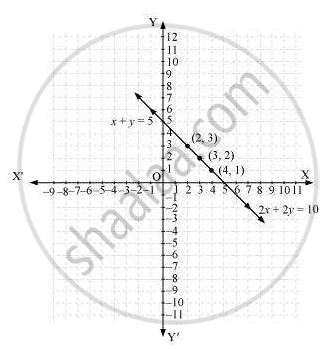Share

# Which of the following pairs of linear equations are consistent/ inconsistent? If consistent, obtain the solution graphically: x + y = 5, 2x + 2y = 10 - CBSE Class 10 - Mathematics

ConceptGraphical Method of Solution of a Pair of Linear Equations

#### Question

Which of the following pairs of linear equations are consistent/ inconsistent? If consistent, obtain the solution graphically: x + y = 5, 2x + 2y = 10

#### Solution

x + y = 5

2x + 2y = 10

a_1/a_2=1/2, b_1/b_2=1/2, c_1/c_2 = 5/10 =1/2

Since a_1/a_2=b_1/b_2=c_1/c_2

Therefore, these linear equations are coincident pair of lines and thus have infinite number of possible solutions. Hence, the pair of linear equations is consistent.

x + y = 5

x = 5 - y

 x 4 3 2 y 1 2 3

And, 2x + 2y = 10

x =(10-2y)/2

 x 4 3 2 y 1 2 3

Hence, the graphic representation is as follows.From the figure, it can be observed that these lines are overlapping each other. Therefore, infinite solutions are possible for the given pair of equations

Is there an error in this question or solution?

#### APPEARS IN

NCERT Solution for Mathematics Textbook for Class 10 (2019 to Current)
Chapter 3: Pair of Linear Equations in Two Variables
Ex. 3.20 | Q: 4.1 | Page no. 50
Solution Which of the following pairs of linear equations are consistent/ inconsistent? If consistent, obtain the solution graphically: x + y = 5, 2x + 2y = 10 Concept: Graphical Method of Solution of a Pair of Linear Equations.
S